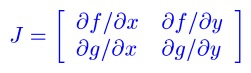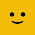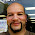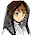## Sunday, September 8, 2013

### Differential Equations #10: Critical Points

Critical Points and Determining What Happens

In this blog entry we are working with a system of two equations:

x' = f(x,y)
y' = g(x,y)

where x and y are functions of a independent variable, say t for example. Well treat t as a time variable.

Today's blog will cover a three step process:
1. Finding Critical Points
2. Determining the Jacobian Matrix
3. Finding the Eiegenvalues of that Jacobian Matrix

1. Finding Critical Points

It is relatively easy to find the critical points of a system. Add that needs to be done is to set x' = 0 and y' = 0. The next step is to solve for x and y.

2. Determining the Jacobian Matrix

The Jacobian matrix of the system isHint: If the system is
x' = Ax + By
y' = Cx + Dy

Then the system's critical point is (0, 0) and the Jacobian Matrix is

J = [ [A, B] [C, D] ]

3. Determine the Eigenvalues

As we recall the eigenvalues of a 2 × 2 matrix can be determined by solving the following characteristic equation:

λ^2 - trace * λ + determinant = 0

Where:
* trace is the sum of the matrix's diagonal elements
* determinant is the determinant of the matrix

The roots are crucial and determine the behavior of the critical point.

Types of critical points:
* source: individual curve solutions x(t) and y(t) are trajectories going away from the critical point
* sink: individual curve solutions x(t) and y(t) are trajectories go towards the critical point
* saddle point: the critical point acts as a sink for some trajectories and a source for other trajectories
* center: trajectories orbit around the critical point, most likely circular or elliptical orbits

Determining the type of critical points:
* λ1 and λ2 are real and positive: critical point is a source.
* λ1 and λ2 are real and negative: critical point is a sink
* λ1 and λ2 are real and have opposite signs: critical point is a saddle
* λ is a double root and it's positive: critical point is a source
* λ is a double root and it's negative: critical point is a sink
* λ = S ± Ti and S is positive: point is a source, trajectories are spiral
* λ = S ± Ti and S is negative: point is a sink, trajectories are spiral
* λ = ± Ti: critical point is a center

Source: http://kaharris.org/teaching/216/Lectures/lec28/lec28.pdf

Let's work with some examples.

1.
x' = x + 4y
y' = 2x + 3y

Critical Point:
0 = x + 4y
0 = 2x + 3y

x = -4y
0 = 2(-4y) + 3y which implies y = 0 and x = 0

Our critical point is (0,0). The Jacobian matrix is J = [ [1, 4] [2, 3] ].

trace(J) = 1 + 3 = 4
det(J) = 1 * 3 - 2 * 4 = -5

The eigenvalues are:
λ^2 - 4*λ - 5 = 0
(λ - 5)(λ + 1) = 0
λ1 = 5, λ2 = -1
Real and opposite signs, the critical point (0,0) is a saddle point.

2.
x' = x + 2y - 6
y' = 6x - 3y + 24

Critical Point:
0 = x + 2y - 6
0 = 6x - 3y + 24

Which leaves is with the system of
x + 2y = 6
6x - 3y = -24

Solving the system yields the critical point (-2, 4).

With
f = x + 2y - 6
df/dx = 1
df/dy = 2
g = 6x - 3y + 24
dg/dx = 6
dg/dy = -3,

The Jacobian matrix is
J = [ [1, 2] [6, -3] ]

The eigenvalues of J are 3 and -5. Since the eigenvalues are real and are of opposite signs, the critical point (-2, 4) is a saddle point.

3.
x' = 2x + 3y
y' = x - 2y^2

Finding the critical points:
0 = 2x + 3y
0 = x - 2y^2

x = 2y^2
0 = 2(2y^2) + 3y
0 = 4y^2 + 3y
0 = y * (4y + 3)
which implies that we have two critical points, one with y = 0 and the other with y = -3/4.

If y = 0, then x = 2*0^2 = 0. One critical point is (0,0).
If y = -3/4, then x = 2*(-3/4)^2 = 9/8. The other critical point is (9/8, -3/4).

With
f = 2x + 3y, df/dx = 2, df/dy = 3
g = x - 2y^2, dg/dx = 1, dg/dy = -4y

Critical Point (0,0):
J = [ [2, 3] [1, 0] ]
And the eigenvalues of J are -1 and 3.
The critical point (0,0) is a saddle point.

Critical Point (9/8, -3/4):
J = [ [2, 3] [1, 3] ]
And the eigenvalues are (5 ± √13)/2. Approximate values are 4.302776 and 0.697224. The critical point (9/8, -3/4) is a source.

Hope this helps and we'll talk next time. Please feel free to post questions and comments.

Eddie

This blog is property of Edward Shore. 2013

1.Thank You So Much! This article was of a great help ! :)

1.Thank you Geetika! Glad to be of service. :)

Eddie

2.Excellent article. Well written, concise and totally understandable. Thanks

3.Excellent, thank you!

4.5.Very thorough, thank you!

6.Very easy to understanding. Thank you so much. but you can use an graphical representation for source, sink, saddle point, center for better understanding.

### Retro Review: Radio Shack EC-2024

Retro Review:  Radio Shack EC-2024 Quick Facts Company:  Radio Shack Years in Production: Around 1994 Original Price: \$24.99 Bat...